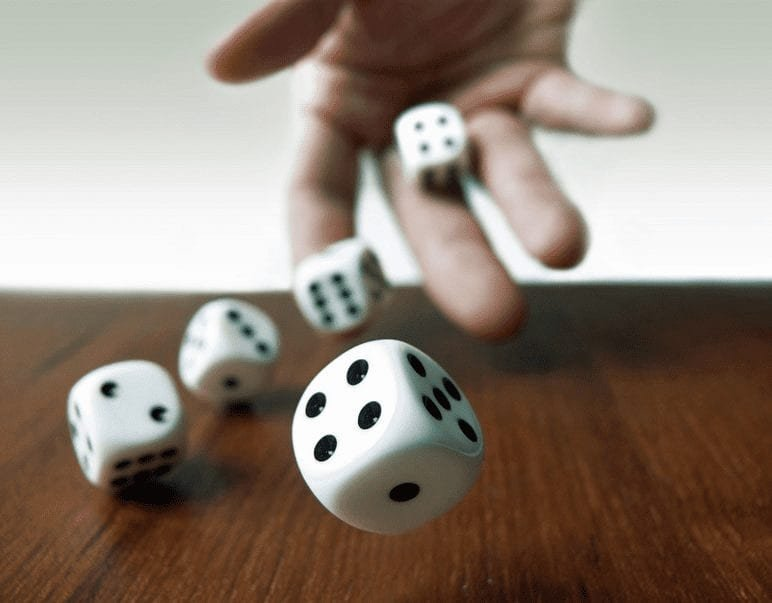Get inspired by the success stories of our students in IIT JAM MS, ISI  MStat, CMI MSc Data Science.  Learn More

# How to roll a Dice by tossing a Coin ? Cheenta Statistics DepartmentHow can you roll a dice by tossing a coin? Can you use your probability knowledge? Use your conditioning skills.

Suppose, you have gone to a picnic with your friends. You have planned to play the physical version of the Snake and Ladder game. You found out that you have lost your dice.

The shit just became real!

Now, you have an unbiased coin in your wallet / purse. You know Probability.

### Aapna Time Aayega

starts playing in the background. :p

## Can you simulate the dice from the coin?

Ofcourse, you know chances better than others. :3

Take a coin.

Toss it 3 times. Record the outcomes.

HHH = Number 1

HHT = Number 2

HTH = Number 3

HTT = Number 4

THH = Number 5

THT = Number 6

TTH = Reject it, don't ccount the toss and toss again

TTT = Reject it, don't ccount the toss and toss again

Voila done!

What is the probability of HHH in this experiment?

Let X be the outcome in the restricted experiment as shown.

How is this experiment is different from the actual experiment?

This experiment is conditioning on the event A = {HHH, HHT, HTH, HTT, THH, THT}.

$P( X = HHH) = P (X = HHH | X \in A ) = \frac{P (X = HHH)}{P (X \in A)} = \frac{1}{6}$

Beautiful right?

Can you generalize this idea?

## Food for thought

• Give an algorithm to simulate any conditional probability.
• Give an algorithm to simulate any event with probability $\frac{m}{2^k}$, where $m \leq 2^k$.
• Give an algorithm to simulate any event with probability $\frac{m}{2^k}$, where $n \leq 2^k$.
• Give an algorithm to simulate any event with probability $\frac{m}{n}$, where $m \leq n \leq 2^k$ using conditional probability.

## Watch the Video here:

Books for ISI MStat Entrance Exam

How to Prepare for ISI MStat Entrance Exam

ISI MStat and IIT JAM Stat Problems and Solutions

Cheenta Statistics Program for ISI MStat and IIT JAM Stat

Simple Linear Regression - Playlist on YouTube

How can you roll a dice by tossing a coin? Can you use your probability knowledge? Use your conditioning skills.

Suppose, you have gone to a picnic with your friends. You have planned to play the physical version of the Snake and Ladder game. You found out that you have lost your dice.

The shit just became real!

Now, you have an unbiased coin in your wallet / purse. You know Probability.

### Aapna Time Aayega

starts playing in the background. :p

## Can you simulate the dice from the coin?

Ofcourse, you know chances better than others. :3

Take a coin.

Toss it 3 times. Record the outcomes.

HHH = Number 1

HHT = Number 2

HTH = Number 3

HTT = Number 4

THH = Number 5

THT = Number 6

TTH = Reject it, don't ccount the toss and toss again

TTT = Reject it, don't ccount the toss and toss again

Voila done!

What is the probability of HHH in this experiment?

Let X be the outcome in the restricted experiment as shown.

How is this experiment is different from the actual experiment?

This experiment is conditioning on the event A = {HHH, HHT, HTH, HTT, THH, THT}.

$P( X = HHH) = P (X = HHH | X \in A ) = \frac{P (X = HHH)}{P (X \in A)} = \frac{1}{6}$

Beautiful right?

Can you generalize this idea?

## Food for thought

• Give an algorithm to simulate any conditional probability.
• Give an algorithm to simulate any event with probability $\frac{m}{2^k}$, where $m \leq 2^k$.
• Give an algorithm to simulate any event with probability $\frac{m}{2^k}$, where $n \leq 2^k$.
• Give an algorithm to simulate any event with probability $\frac{m}{n}$, where $m \leq n \leq 2^k$ using conditional probability.

## Watch the Video here:

Books for ISI MStat Entrance Exam

How to Prepare for ISI MStat Entrance Exam

ISI MStat and IIT JAM Stat Problems and Solutions

Cheenta Statistics Program for ISI MStat and IIT JAM Stat

Simple Linear Regression - Playlist on YouTube

This site uses Akismet to reduce spam. Learn how your comment data is processed.

### One comment on “How to roll a Dice by tossing a Coin ? Cheenta Statistics Department”

1.user says:

nice way!!!!!!!!!!!!!!!!

### Knowledge Partner